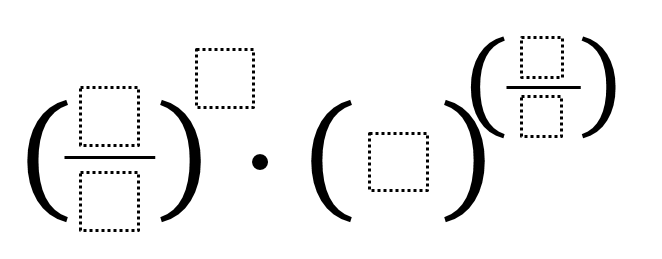# Rational Exponents

Directions: Using the digits 1 to 6, at most one time each, fill in the boxes make the greatest or least value. Extension: How close to 1 can you get?### Hint

Which of the boxes should get the largest number? Which should get the smallest number?

There are 720 (6!) total possible arrangements of the numbers 1-6 in this equation… do you have to check them all?

The largest number is 140625 = (5/1)^(6) * 3^(4/2)
The smallest number is 0.0001076 = (1/5)^6 * 2^(3/4)
The closest to 1 is 1.01047 = (4/6)^1 * 2^(3/5)

Source: Erick Lee

## Imaginary Solutions to a Quadratic Equation

Directions: Using the digits 1 to 9 at most one time each, place a digit …

1.•2.